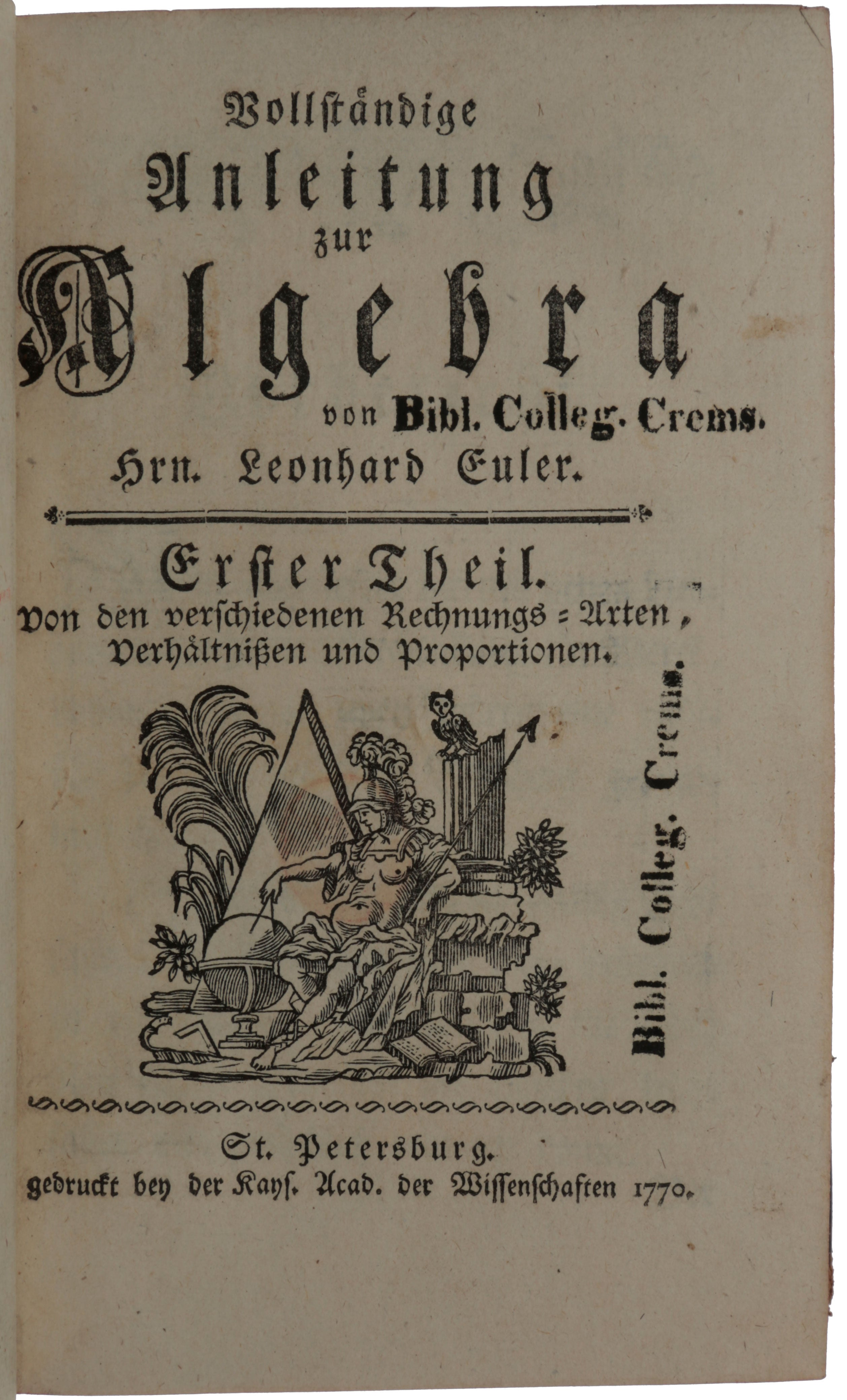Recently found by viaLibri....
2020-11-14 16:08:41EULER, Leonhard
Vollständige Anleitung zur Algebra. Erster [- Zweiter] Theil
Imperial Academy of Sciences, St. Petersburg, 1770. THE MOST SUCCESSFUL MATHEMATICS TEXTBOOK SINCE EUCLID. First edition in German, its language of composition, and first obtainable edition, of Euler's 'Complete instruction in algebra', which takes the reader step-by-step from the basics, including negative and imaginary numbers, through to the solution of quadratic, cubic and biquadratic equations, and concluding with a long section on Diophantine problems (i.e., the solution of algebraic equations in whole numbers). "In 1770 Euler published his Vollständige Anleitung zur Algebra . . . The first volume treats of determinate algebra. This contains one of the earliest attempts to place the fundamental processes on a scientific basis: the same subject had attracted D'Alembert's attention. This work also includes the proof of the binomial theorem for an unrestricted real index which is still known by Euler's name . . . The second volume of the 'Algebra' treats of indeterminate or Diophantine algebra. This contains the solutions of some of the problems proposed by Fermat, and which had hitherto remained unsolved" (Rouse Ball, A Short Account of the History of Mathematics, p. 397). "The entire second section of volume 2 was devoted to Diophantine analysis; it gave his proof of Fermat's last theorem for the case n = 3 [i.e., that the sum of two cubes of whole numbers cannot be a cube of a whole number]" (Calinger, Leonhard Euler, pp. 477-8). Euler's proof, "based on the method of infinite descent and using complex numbers of the form a + b?-3, is thoroughly described in his Volls … [Click Below for Full Description]
Bookseller: SOPHIA RARE BOOKS [Koebenhavn V, Denmark]
Check availability: